In a recent blog post, Chris Hemedinger used a scatter plot to show the result of 100 coin tosses. Chris arranged the 100 results in a 10 x 10 grid, where the first 10 results were shown on the first row, the second 10 were shown on the second row, and so on. Placing items along each row before going to the next row is called row-major order.

An implicit formula for arranging items in rows

If you process items sequentially, it is easy to position the items in a grid by using an inductive scheme:

1. Place the first item at (1, 1).
2. Assume the n_th is placed at position (r, c). Place the (n+1)st item at position (r, c+1) if there is room on the current row, otherwise place it at (r+1, 1), which is the first element of the next row.

The inductive scheme is also called an implicit or recursive formula because the position of the (n+1)st item is given in terms of the position of the nth item.

For example, suppose that you have 70 items and you want to place 11 items in each row. The inductive algorithm looks like the following:

```%let Nx = 11; /* number of items in row */ data Loc; label r = "Row" c = "Column"; retain r 1 c 1 item 1; output; /* base case */ do item = 2 to 70; /* inductive step */ c + 1; if c > &Nx then do; r + 1; c = 1; end; output; end; run;   title "Position of Items in Grid"; proc sgplot data=Loc; text x=c y=r text=item / textattrs=(size=12) position=center strip; xaxis integer offsetmin=0.05 offsetmax=0.05; yaxis reverse offsetmin=0.05 offsetmax=0.05; run;```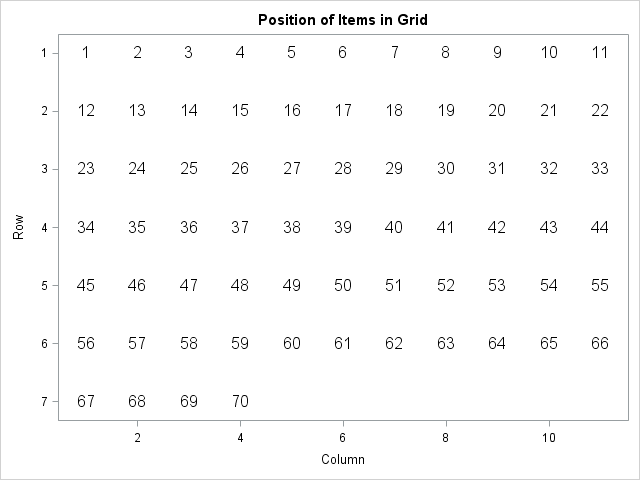The inductive algorithm is easy to implement and to understand. However, it does not enable you to easily determine the row and column of the 1,234,567_th item if there are 11 items in each row. Nor does it enable you to compute the positions when the index increments by a value greater than 1. To answer these questions, you need to use an explicit or direct formula.

An explicit formula for arranging items in rows

The explicit formula uses the MOD function to compute the column position and integer division to compute the row position. SAS does not have an explicit "integer division operator," but you can emulate it by using the FLOOR function. The following macro definitions encapsulate the formulas:

```/* (row, col) for item n if there are Nx items in each row (count from 1), assuming row-major order */ %macro ColPos(n, Nx); 1 + mod(&n.-1, &Nx.) %mend; %macro RowPos(n, Nx); 1 + floor((&n.-1) / &Nx.) %mend;```

The formulas might look strange because they subtract 1, do a calculation, and then add 1. This formula assumes that you want to count the items, rows, and columns beginning with 1. If you prefer to count from 0 then the formulas become MOD(n, Nx) and FLOOR(N/Nx).

You can use the formulas to directly compute the position of the odd integers in the digits 1–70 when there are 11 items on each row:

```%let Nx = 11; data grid; do item = 1 to 70 by 2; /* only odd integers */ row = %RowPos(item, &Nx); col = %ColPos(item, &Nx); output; end; run;   title "Position of Odd Integers in Grid"; proc sgplot data=grid; text x=col y=row text=item / textattrs=(size=12) position=center strip; xaxis integer offsetmin=0.05 offsetmax=0.05 label="Column" max=&Nx; yaxis reverse offsetmin=0.05 offsetmax=0.05 integer label="Row"; run;```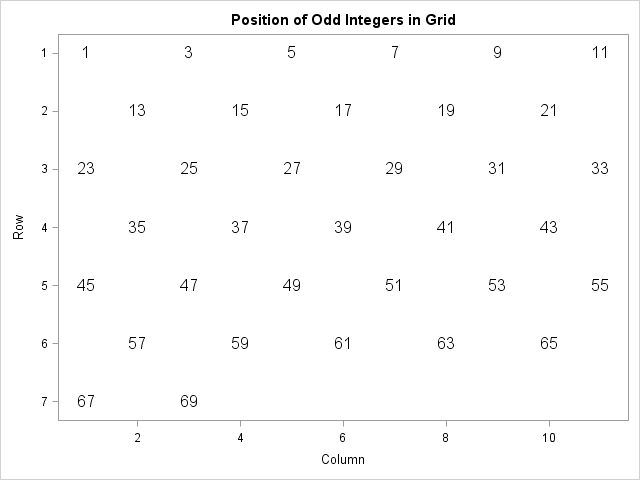Of course, you can also use the direct formula to process items incrementally. The following DATA step computes the positions for 19 observations in the Sashelp.Class data set, where five names are placed in each row:

```data gridName; set sashelp.class; y = %RowPos(_N_, 5); /* 5 columns in each row */ x = %ColPos(_N_, 5); run;   title "Position Five Names in Each Row"; proc sgplot data=gridName; text x=x y=y text=Name / textattrs=(size=12) position=center strip; xaxis integer offsetmin=0.08 offsetmax=0.08; yaxis reverse offsetmin=0.05 offsetmax=0.05 integer; run;```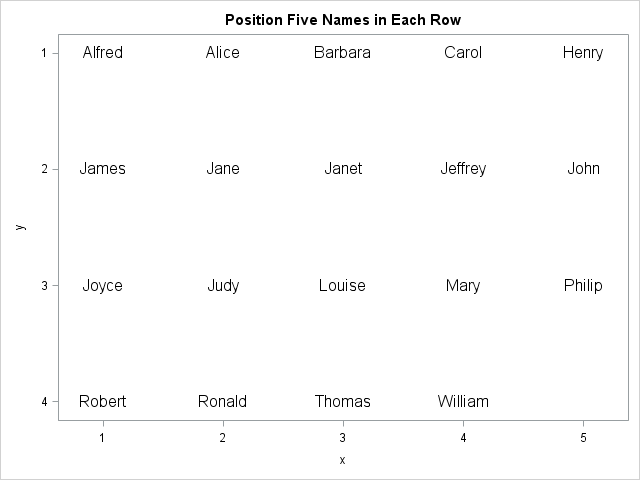The explicit formula is used in the SAS/IML NDX2SUB function, which tells you the row and column information for the n_th item in a matrix.

In summary, you can use an implicit formula or an explicit formula to arrange items in rows, where each row contains Nx items. The implicit formula is useful when you are arranging the items sequentially. The explicit formula is ideal when you are randomly accessing the items and you need a direct computation that provides the row and column position.

Finally, if you want to arrange items in column-major order (down the first column, then down the second,...), you can use similar formulas. The row position of the n_th item is 1 + mod(n-1, Ny) and the column position is 1 + floor((n-1) / Ny), where Ny is the number of rows in the grid.

The post Position items in a grid appeared first on The DO Loop.Datasets can present themselves in different ways. Identical data can bet arranged differently, often as wide or tall datasets. Generally, the tall dataset is better. Learn how to convert wide data into tall data with PROC TRANSPOSE.

The post "Wide" versus "Tall" data: PROC TRANSPOSE v. the DATA step appeared first on SAS Learning Post.

Do you periodically delete unneeded global macro variables? You should! Deleting macro variables releases memory and keeps your symbol table clean. Learn how the macro language statement that deletes global macro variables and about the %DELETEALL statement that can be a life saver for macro programmers.

The post Deleting global macro variables appeared first on SAS Learning Post.

SAS variables are variables in the statistics sense, not the computer programming sense. SAS has what many computer languages call “variables,” it just calls them “macro variables.” Knowing the difference between SAS variables and SAS macro variables will help you write more flexible and effective code.

The post When a variable is not a variable appeared first on SAS Learning Post.My article about the difference between CLASS variables and BY variables in SAS focused on SAS analytical procedures. However, the BY statement is also useful in the SAS DATA step where it is used to merge data sets and to analyze data at the group level. When you use the BY statement in the DATA step, the DATA step creates two temporary indicator variables for each variable in the BY statement. The names of these variables are FIRST.variable and LAST.variable, where variable is the name of a variable in the BY statement. For example, if you use the statement BY Sex, then the names of the indicator variables are FIRST.Sex and LAST.Sex.

This article gives several examples of using the FIRST.variable and LAST.variable indicator variables for BY-group analysis in the SAS DATA step. The first example shows how to compute counts and cumulative amounts for each BY group. The second example shows how to compute the time between the first and last visit of a patient to a clinic, as well as the change in a measured quantity between the first and last visit. BY-group processing in the DATA step is a fundamental operation that belongs in every SAS programmer's tool box.

Use FIRST. and LAST. variables to find count the size of groups

The first example uses data from the Sashelp.Heart data set, which contains data for 5,209 patients in a medical study of heart disease. The data are distributed with SAS. The following DATA step extracts the Smoking_Status and Weight variables and sorts the data by the Smoking_Status variable:

```proc sort data=Sashelp.Heart(keep=Smoking_Status Weight) out=Heart; by Smoking_Status; run;```

Because the data are sorted by the Smoking_Status variable, you can use the FIRST.Smoking_Status and LAST.Smoking_Status temporary variables to count the number of observations in each level of the Smoking_Status variable. (PROC FREQ computes the same information, but does not require sorted data.) When you use the BY Smoking_Status statement, the DATA step automatically creates the FIRST.Smoking_Status and LAST.Smoking_Status indicator variables. As its name implies, the FIRST.Smoking_Status variable has the value 1 for the first observation in each BY group and the value 0 otherwise. (More correctly, the value is 1 for the first record and for records for which the Smoking_Status variable is different than it was for the previous record.) Similarly, the LAST.Smoking_Status indicator variable has the value 1 for the last observation in each BY group and 0 otherwise.

The following DATA step defines a variable named Count and initializes Count=0 at the beginning of each BY group. For every observation in the BY group, the Count variable is incremented by 1. When the last record in each BY group is read, that record is written to the Count data set.

```data Count; set Heart; /* data are sorted by Smoking_Status */ BY Smoking_Status; /* automatically creates indicator vars */ if FIRST.Smoking_Status then Count = 0; /* initialize Count at beginning of each BY group */ Count + 1; /* increment Count for each record */ if LAST.Smoking_Status; /* output only the last record of each BY group */ run;   proc print data=Count noobs; format Count comma10.; var Smoking_Status Count; run;```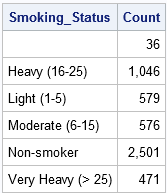The same technique enables you to accumulate values of a variable within a group. For example, you can accumulate the total weight of all patients in each smoking group by using the following statements:

```if FIRST.Smoking_Status then cumWt = 0; cumWt + Weight;```

This same technique can be used to accumulate revenue from various sources, such as departments, stores, or regions.

Use FIRST. and LAST. variables to compute duration of treatment

Another common use of the FIRST.variable and LAST.variable indicator variables is to determine the length of time between a patient's first visit and his last visit. Consider the following DATA step, which defines the dates and weights for four male patients who visited a clinic as part of a weight-loss program:

```data Patients; informat Date date7.; format Date date7. PatientID Z4.; input PatientID Date Weight @@; datalines; 1021 04Jan16 302 1042 06Jan16 285 1053 07Jan16 325 1063 11Jan16 291 1053 01Feb16 299 1021 01Feb16 288 1063 09Feb16 283 1042 16Feb16 279 1021 07Mar16 280 1063 09Mar16 272 1042 28Mar16 272 1021 04Apr16 273 1063 20Apr16 270 1053 28Apr16 289 1053 13May16 295 1063 31May16 269 ;```

For these data, you can sort by the patient ID and by the date of visit. After sorting, the first record for each patient contains the first visit to the clinic and the last record contains the last visit. You can subtract the patient's weight for these dates to determine how much the patient gained or lost during the trial. You can also use the INTCK function to compute the elapsed time between visits. If you want to measure time in days, you can simply subtract the dates, but the INTCK function enables you to compute duration in terms of years, months, weeks, and other time units.

```proc sort data=Patients; by PatientID Date; run;   data weightLoss; set Patients; BY PatientID; retain startDate startWeight; /* RETAIN the starting values */ if FIRST.PatientID then do; startDate = Date; startWeight = Weight; /* remember the initial values */ end; if LAST.PatientID then do; endDate = Date; endWeight = Weight; elapsedDays = intck('day', startDate, endDate); /* elapsed time (in days) */ weightLoss = startWeight - endWeight; /* weight loss */ AvgWeightLoss = weightLoss / elapsedDays; /* average weight loss per day */ output; /* output only the last record in each group */ end; run;   proc print noobs; var PatientID elapsedDays startWeight endWeight weightLoss AvgWeightLoss; run;```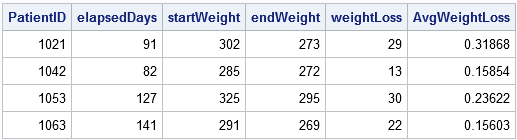The output data set summarizes each patient's activities at the clinic, including his average weight loss and the duration of his treatment.

Some programmers think that the FIRST.variable and LAST.variable indicator variables require that the data be sorted, but that is not true. The temporary variables are created whenever you use a BY statement in a DATA step. You can use the NOTSORTED option on the BY statement to process records regardless of the sort order.

Summary

In summary, the BY statement in the DATA step automatically creates two indicator variables. You can use the variables to determine the first and last record in each BY group. Typically the FIRST.variable indicator is used to initialize summary statistics and to remember the initial values of measurement. The LAST.variable indicator is used to output the result of the computations, which often includes simple descriptive statistics such as a sum, difference, maximum, minimum, or average values.

BY-group processing in the DATA step is a common topic that is presented at SAS conferences. Some authors use FIRST.BY and LAST.BY as the name of the indicator variables. For further reading, I recommend the paper "The Power of the BY Statement" (Choate and Dunn, 2007). SAS also provides several samples about BY-group processing in the SAS DATA step, including the following:

The post How to use FIRST.variable and LAST.variable in a BY-group analysis in SAS appeared first on The DO Loop.When I first learned to program in SAS, I remember being confused about the difference between CLASS statements and BY statements. A novice SAS programmer recently asked when to use one instead of the other, so this article explains the difference between the CLASS statement and BY variables in SAS procedures.

The BY statement and the CLASS statement in SAS both enable you to specify one or more categorical variables whose levels define subgroups of the data. (For simplicity, we consider only a single categorical variable.) The primary difference is that the BY statement computes many analyses, each on a subset of the data, whereas the CLASS statement computes a single analysis of all the data. Specifically,

• The BY statement repeats an analysis on every subgroup. The subgroups are treated as independent samples. If a BY variable defines k groups, the output will contains k copies of every table and graph, one copy for the first group, one copy for the second group, and so on.
• The CLASS statement enables you to include a categorical variable as part of an analysis. Often the CLASS variable is used to compare the groups, such as in a t test or an ANOVA analysis. In regression models, the CLASS statement enables you to estimate parameters for the levels of a categorical variable, thereby estimating the effect of each level on the response. Another use of a CLASS variable is to define categories for a classification task, such as a discriminant analysis.

To illustrate the differences between an analysis that uses a BY statement and one that uses a CLASS statement, let's create a subset (called Cars) of the Sashelp.Cars data. The levels of the Origin variable indicate whether a vehicle is manufactured in "Asia", "Europe", or the "USA". For efficiency reasons, most classical SAS procedures require that you sort the data when you use a BY statement. Therefore, a call to PROC SORT creates a sorted version of the data called CarsSorted, which will be used for the BY-group analyses.

```data Cars; set Sashelp.Cars; where cylinders in (4,6,8) and type ^= 'Hybrid'; run;   proc sort data=Cars out=CarsSorted; by Origin; run;```

Descriptive statistics for grouped data

When you generate descriptive statistics for groups of data, the univariate statistics are identical whether you use a CLASS statement or a BY statement. What changes is the way that the statistics are displayed. When you use the CLASS statement, you get one table that contains all statistics or one graph that shows the distribution of each subgroup. However, when you use the BY statement you get multiple tables and graphs.

The following statements use the CLASS statement to produce descriptive statistics. PROC UNIVARIATE displays one (paneled) graph that shows a comparative histogram for the vehicles that are made in Asia, Europe, and USA. PROC MEANS displays one table that contains descriptive statistics:

```proc univariate data=Cars; class Origin; var Horsepower; histogram Horsepower / nrows=3; /* must use NROWS= to get panel */ ods select histogram; run;   proc means data=Cars N Mean Std; class Origin; var Horsepower Weight Mpg_Highway; run;```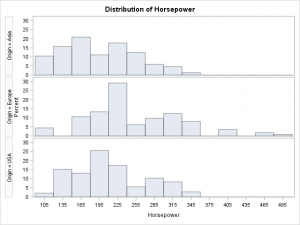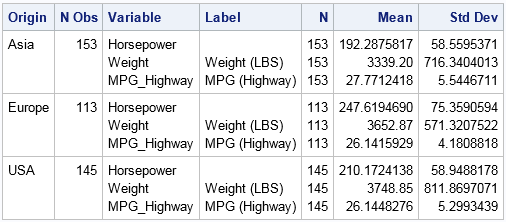In contrast, if you run a BY-group analysis on the levels of the Origin variable, you will see three times as many tables and graphs. Each analysis is preceded by a label that identifies each BY group. Notice that the BY-group analysis uses the sorted data.

```proc means data=CarsSorted N Mean Std; by Origin; var Horsepower Weight Mpg_Highway; run;```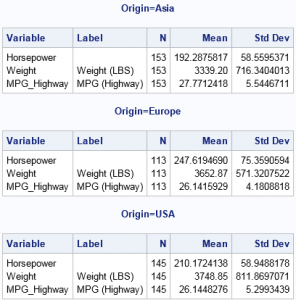Always remember that the output from a BY statement is equivalent to the output from running the procedure multiple times on subsets of the data. For example, the previous statistics could also be generated by calling PROC MEANS three times, each call with a different WHERE clause, as follows:

```proc means N Mean Std data=CarsSorted( where=(origin='Asia') ); var Horsepower Weight Mpg_Highway; run; proc means N Mean Std data=CarsSorted( where=(origin='Europe') ); var Horsepower Weight Mpg_Highway; run; proc means N Mean Std data=CarsSorted( where=(origin='USA') ); var Horsepower Weight Mpg_Highway; run;```

In fact, if you ever find yourself repeating an analysis many times (perhaps by using a macro loop), you should consider whether you can rewrite your program to be more efficient by using a BY statement.

Comparing groups: Use the CLASS statement

As a general rule, you should use a CLASS statement when you want to compare or contrast groups. For example, the following call to PROC GLM performs an ANOVA analysis on the horsepower (response variable) for the three groups defined by the Origin variable. The procedure automatically creates a graph that displays three boxplots, one for each group. The procedure also computes parameter estimates for the levels of the CLASS variable (not shown).

```proc glm data=Cars; /* by default, create graph with side-by-side boxplots */ class Origin; model Horsepower = Origin / solution; run;```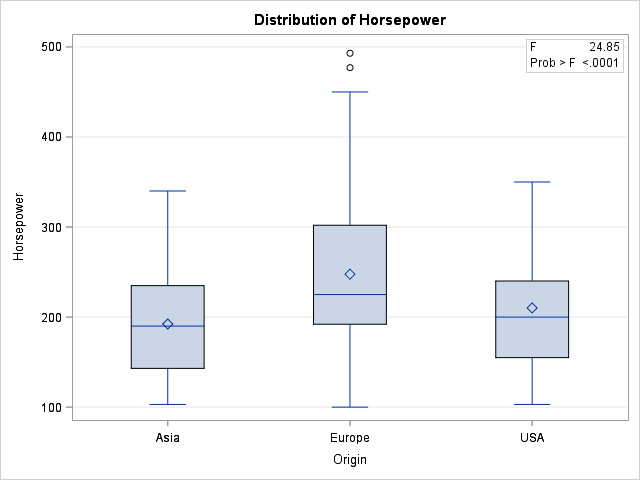You can specify multiple variables on the CLASS statement to include multiple categorical variables in a model. Any variables that are not listed on the CLASS statement are assumed to be continuous. Thus the following call to PROC GLM analyzes a model that has one continuous and one classification variable. The procedure automatically produces a graph that overlays the three regression curves on the data:

```ods graphics /antialias=on; title "CLASS Variable Regression: One Model with Multiple Parameters"; proc GLM data=Cars plots=FitPlot; class Origin; model Horsepower = Origin | Weight / solution; ods select ParameterEstimates ANCOVAPlot; quit;```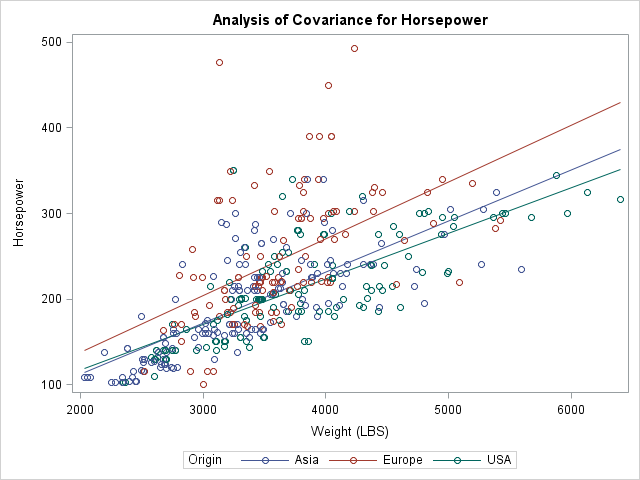In contrast, if you use a BY statement, the Origin variable cannot be part of the model but is used only to subset the data. If you use a BY statement, you obtain three different models of the form Horsepower = Weight. You get three parameter estimates tables and three graphs, each showing one regression line overlaid on a subset of the data.

Predicted Values: CLASS VARIABLE versus BY Variable

When you use a BY statement and fit three models of the form Horsepower = Weight, the procedure fits a total of six parameters. Notice that when you use the CLASS statement and fit the model Horsepower = Origin | Weight, you also fit six free parameters. It turns out that these two methods produce the same predicted values. In fact, you can combine the parameter estimates (for the GLM parameterization) for the CLASS model to obtain the parameter estimates from the BY-variable analysis, as shown below. Each parameter estimate for the BY-variable models are obtained as the sum of two estimates for the CLASS-variable analysis: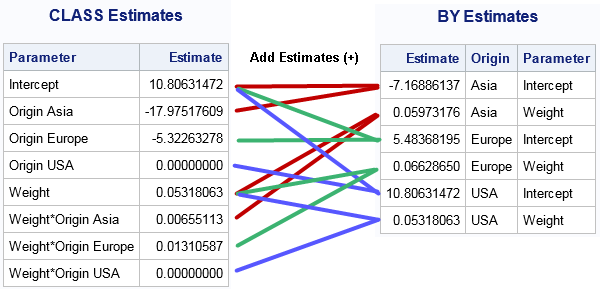For many regression models, the predicted values for the BY-variable analyses are the same as for a particular model that uses a CLASS variable. As shown above, you can even see how the parameters are related when you use a GLM or reference parameterization. However, the CLASS variable formulation can fit models (such as the equal-slope model Horsepower = Origin Weight) that are not available when you use a BY variable to fit three separate models. Furthermore, the CLASS statement provides parameter estimates so that you can see the effect of the groups on the response variable. It is more difficult to compare the models that are produced by using the BY statement.

Other CLASS-like statements in SAS

Some SAS procedures use other syntax to analyze groups. In particular, the SGPLOT procedure calls classification variables "group variables." If you want to overlay graphs for multiple groups, you can use the GROUP= option on many SGPLOT statements. (Some statements support the CATEGORY= option, which is similar.) For example, to replicate the two-variable regression analysis from PROC GLM, you can use the following statements in PROC SGPLOT:

```proc sgplot data=Cars; reg y=Horsepower x=Weight / group=Origin; /* Horsepower = Origin | Weight */ run;```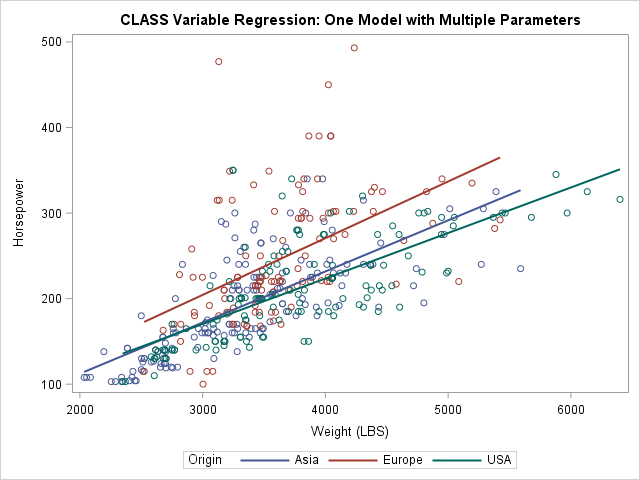Summary

In summary, use the BY statement in SAS procedures when you want to repeat an analysis for every level of one or more categorical variables. The variables define the subsets but are not otherwise part of the analysis. In classical SAS procedures, the data must be sorted by the BY variables. A BY-group analysis can produce many tables and graphs, so you might want to suppress the ODS output and write the results to a SAS data set.

Use the CLASS statement when you want to include a categorical variable in a model. A CLASS statement often enables you to compare or contrast subgroups. For example, in regression models you can evaluate the relative effect of each level on the response variable.

In some cases, the BY statement and the CLASS statement produce identical statistics. However, the CLASS statement enables you to fit a wider variety of models.

The post The difference between CLASS statements and BY statements in SAS appeared first on The DO Loop.Want to see my newly minted certified professional badge? Scroll down to take a peek. Yes, I managed to successfully complete the Base SAS Programmer certification exam… with, ahem, flying colors I might add. Here are my tips to tackle the Base SAS certification exam: 1.  Get clear on the [...]

The post Demystifying certification (Part 3): To the finish appeared first on SAS Learning Post.

Do you know what the #1 fear in North America is? Most people say fear of public speaking or fear of death, but you may just want to consider this new fear upping the charts - the fear of writing a SAS Certification exam! The real test begins before even [...]

The post Demystifying SAS Certification (Part 2): Write it down appeared first on SAS Learning Post.If Necessity is the mother of Invention, then, perhaps, the father of Automation is Laziness. Automation is all about convenience, comfort, and productivity. Why do it yourself if you can devise something to do it for you!

In my previous post Running SAS programs in batch under Unix/Linux, we learned that in order to automate SAS jobs submissions, they are often run in batch mode. We also learned that we usually create batch scripts as a convenient way to run SAS programs in batch. To create a unique SAS log file generated with each batch submission, a typical batch script may look like follows:

```#!/usr/bin/sh dtstamp=\$(date +%Y.%m.%d_%H.%M.%S) pgmname="/sas/code/project1/program1.sas" logname="/sas/code/project1/program1_\$dtstamp.log" /sas/SASHome/SASFoundation/9.4/sas \$pgmname -log \$logname```

It will allow you to submit your SAS program /sas/code/project1/program1.sas in batch, and also capture SAS log file with a convenient date-time suffix in the same directory.

SAS program to write batch scripts

But what if we are deploying multiple SAS programs? Well, then we would need to create a batch script for each of them. They will all look similar to each other, and that is when most human errors usually occur – when we do something similar, monotonously, over and over again.  Besides, I found working with the Unix Visual Editor (“vi editor”) is not quite a 21st century experience.

What would a normal SAS programmer do in such a situation? That’s right – we would write a SAS program to write a batch script file! Let’s do it. Let’s automate the automation.

In its simplest form, to replicate the above batch script example our SAS program would look like this:

```filename b '/sas/code/project1/program1.sh';   data _null_; file b; input; put _infile_; datalines; #!/usr/bin/sh dtstamp=\$(date +%Y.%m.%d_%H.%M.%S) pgmname="/sas/code/project1/program1.sas" logname="/sas/code/project1/program1_\$dtstamp.log" /sas/SASHome/SASFoundation/9.4/sas \$pgmname -log \$logname ;```

Setting up batch file permissions

As we already know from my previous post, we need to assign certain permissions to our batch file in order to make it executable. For example, if you want to give yourself (Owner) and Group execution permissions then your script file permissions can be as:

-rwxr-x---, or 750 in octal representation.

In order to do that you can to add to your SAS code the following x-statement:

```options noxwait; x 'chmod 750 /sas/code/project1/program1.sh';```

Alternatively, you can use %SYSEXEC macro statement (no quoting for the OS command) or SYSTASK statement, or CALL SYSTEM routine (used within a data step).

When you create a batch script by running the above code in SAS Enterprise Guide (EG), you don’t have to leave the comfort of your SAS environment or even touch Unix vi editor. Moreover, you can even submit your SAS job in batch mode right from your SAS EG Program Editor.

However, all of these will work fine, unless XCMD System Option is disabled (NOXCMD).

Assigning batch file permissions when XCMD System Option is disabled

`ERROR: Shell escape is not valid in this SAS session.`

Bummer! Have you ever seen this error message in SAS Enterprise Guide while trying to run SAS code with the X statement? It indicates that executing OS commands in the SAS environment is not allowed.

In many organizations, IT department policies do not allow enabling the SAS XCMD system option due to cyber-security concerns. This is usually done system-wide for the whole SAS Enterprise client-server installation via SAS configuration.  In this case, no operating system command is allowed to be executed from within SAS.

Of course, this substantially limits SAS’ automation power, but that is the goal and the price to pay for enhanced security.

Still, even without OS command execution at our disposal, we can set Unix script file permissions using FILENAME statement’s PERMISSION= option. Then our above filename statement will look like this:

```filename b '/sas/code/project1/program1.sh' permission='A::u::rwx,A::g::r-x,A::o::---';```

However, it is important to realize that your ability to fully control file permissions via FILENAME statement’s PERMISSION= option is still restricted by the Unix umask value set by your IT system administrator. But usually, it is not overly restrictive, at least for the purpose of creating executable files in the environments I have worked with.

The double benefit of the FILENAME statement’s PERMISSION= option is that it can be used for setting up file permissions in any SAS installation whether the XCMD system option is enabled or disabled.

SAS macro to create batch script files

Let’s wrap all the above SAS code pieces into a SAS macro that writes batch scripts. Here is the macro code definition:

```%macro write_shell(code); %let fdir = %substr(&code,1,%sysfunc(findc(&code,/,b)));   options dlcreatedir; libname _flib "&fdir"; libname _flib;   %let core = %substr(&code,1,%eval(%length(&code)-4)); filename _fout "&core..sh" permission='A::u::rwx,A::g::r-x,A::o::---'; data _null_; file _fout; put '#!/bin/sh' // 'now=\$(date +%Y.%m.%d_%H.%M.%S)' / "pgmname=""&code""" / "logname=""&core._\$now.log""" / 'sas \$pgmname -log \$logname' ; run; filename _fout; %mend write_shell;```

The single macro parameter (code) represents full path name of your SAS code. And here is a macro invocation example:

```  %write_shell(/sas/code/project1/program1.sas)```

The assumption here is that the script file gets created in the same directory as the relevant SAS code and SAS logs for each of the batch runs. It will be assigned the same name as your SAS program, only with the .sh name extension. As you can see, we do some string parsing to derive directory name, script file name and SAS log file name from the single macro parameter representing full path name of your SAS code. As an added bonus, if a specified directory (/sas/code/project1/) does not yet exist, it will be created by this macro. DLCREATEDIR System Option (along with the two subsequent libname statements) are responsible for the directory creation.

If you want to create many script files for your multiple SAS programs, you just invoke the macro as many times. You can even go totally data-driven for mass script file creation.

Do you find this useful?

Please let me know in the comments section below if you find this blog post useful. Thank you for reading! I also invite you to share your ideas and experiences on the topic.

Let SAS write batch scripts for you was published on SAS Users.